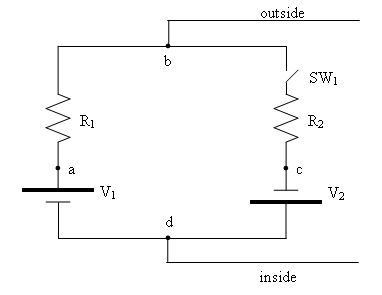Teaching Physics with the Physics Suite Edward F. Redish Problems Sorted by Type | Problems Sorted by Subject | Problems Sorted by Chapter in UP

Modeling a nerve membrane

 (From a homework set in a graduate course in synaptic physiology) As a result of a complex set of biochemical reactions, the cell membrane of a nerve cell pumps ions (Na+ and K+) back and forth across itself, thereby maintaining an electrostatic potential difference from the inside to the outside of the membrane. Modifications of the conditions can result in changes in those potentials. Part of the process can be modeled by treating the membrane as if it were a simple electric circuit consisting of batteries, resistors, and a switch. A simple model of the membrane of a nerve cell is shown in the figure at the right. It consists of two batteries (ion pumps) with voltages V1 = 100 mV and V2 = 50 mV. The resistance to flow across the membrane is represented by two resistors with resistances R1 = 10 kΩ and R2 = 90 kΩ. The variability is represented by a switch, SW1. Four points on the circuit are labelled by the letters a-d. The point b represents the outside of the membrane and the point d the inside of the membrane.1. What is the voltage difference across the membrane (i.e., between d and b) when the switch is open?
2. What is the current flowing around the loop when the switch is closed?
3. What is the voltage drop across the resistor R1 when the switch is open? closed?
4. What is the voltage drop across the resistor R2 when the switch is open? closed?
5. What is the voltage difference across the membrane (i.e., between d and b) when the switch is closed?
6. If the locations of resistances R1 and R2 were reversed would the voltages across the cell membrane be different?